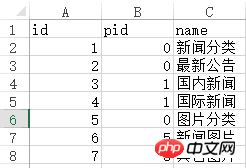# PHP中foreach的用法

foreach有两种语法：

`foreach (array_expression as \$value)`

`foreach (array_expression as \$key => \$value)`

`\$a = array('Tom','Mary','Peter','Jack');`

1、我们用第一种foreach方法来输出。

```foreach (\$a as \$value) {
echo \$value."<br/>";
}```

Tom
Mary
Peter
Jack

2、我们用第二种 foreach 方法来输出。

```foreach (\$a as \$key => \$value) {
echo \$key.','.\$value."<br/>";
}```

0,Tom
1,Mary
2,Peter
3,Jack

`\$b = array('a'=>'Tom','b'=>'Mary','c'=>'Peter','d'=>'Jack');`

```\$b = array(
'a'=>'Tom',
'b'=>'Mary',
'c'=>'Peter',
'd'=>'Jack'
);```

1、我们用第一种foreach方法来输出和上边一样。

```foreach (\$b as \$value) {
echo \$value."<br/>";
}```

Tom
Mary
Peter
Jack

2、我们用第二种foreach方法来输出。

```foreach (\$b as \$key => \$value) {
echo \$key.','.\$value."<br/>";
}```

a,Tom
b,Mary
c,Peter
d,Jack

```\$c = array(
array('1','Tom'),
array('2','Mary'),
array('3','Peter'),
array('4','Jack')
);```

1、我们采用第一种 foreach方法：

```foreach (\$c as \$value) {
print_r(\$value);
echo "<br/>";
}```

Array (  => 11  => Tom )
Array (  => 22  => Mary )
Array (  => 33  => Peter )
Array (  => 44  => Jack )

2、我们采用第二种foreach方法：

```foreach (\$c as \$key => \$value) {
echo '\$key='.\$key."<br/>";
print_r(\$value);
echo "<br/>";
}```

\$key=0
Array (  => 11  => Tom )
\$key=1
Array (  => 22  => Mary )
\$key=2
Array (  => 33  => Peter )
\$key=3
Array (  => 44  => Jack )

```\$d = array(
array('id'=>'11','name'=>'Tom'),
array('id'=>'22','name'=>'Mary'),
array('id'=>'33','name'=>'Peter'),
array('id'=>'44','name'=>'Jack')
);```

1、用第一种方法代码：

```foreach (\$d as \$value) {
print_r(\$value);
echo "<br/>";
}```

Array ( [id] => 11 [name] => Tom )
Array ( [id] => 22 [name] => Mary )
Array ( [id] => 33 [name] => Peter )
Array ( [id] => 44 [name] => Jack )

2、用第二种方法的代码：

```foreach (\$d as \$key => \$value) {
echo '\$key='.\$key."<br/>";
print_r(\$value);
echo "<br/>";
}```

\$key=0
Array ( [id] => 11 [name] => Tom )
\$key=1
Array ( [id] => 22 [name] => Mary )
\$key=2
Array ( [id] => 33 [name] => Peter )
\$key=3
Array ( [id] => 44 [name] => Jack )

```\$d = array(
array('id'=>'11','name'=>'Tom'),
array('id'=>'22','name'=>'Mary'),
array('id'=>'33','name'=>'Peter'),
array('id'=>'44','name'=>'Jack')
);```

```foreach (\$d as \$key => \$value) {
echo (\$value['name']);
echo "<br/>";
}```

```//获取name列作为一维数组
\$nameArr = array(); //name列
foreach (\$d as \$key => \$value) {
\$nameArr[] = \$value['name'];
}
print_r(\$nameArr);```

```Array
(
 => Tom
 => Mary
 => Peter
 => Jack
)```

`\$d = array('Tom','Mary','Peter','Jack');`

```//从数据库中取出的分类数据
\$original_array = array(
array('id' => 1,'pid' => 0,'name' => '新闻分类'),
array('id' => 2,'pid' => 0,'name' => '最新公告'),
array('id' => 3,'pid' => 1,'name' => '国内新闻'),
array('id' => 4,'pid' => 1,'name' => '国际新闻'),
array('id' => 5,'pid' => 0,'name' => '图片分类'),
array('id' => 6,'pid' => 5,'name' => '新闻图片'),
array('id' => 7,'pid' => 5,'name' => '其它图片')
);``````//从数据库中取出的分类数据
\$original_array = array(
array(
'id' => 1,
'pid' => 0,
'name' => '新闻分类'
),
array(
'id' => 2,
'pid' => 0,
'name' => '最新公告'
),
array(
'id' => 3,
'pid' => 1,
'name' => '国内新闻'
),
array(
'id' => 4,
'pid' => 1,
'name' => '国际新闻'
),
array(
'id' => 5,
'pid' => 0,
'name' => '图片分类'
),
array(
'id' => 6,
'pid' => 5,
'name' => '新闻图片'
),
array(
'id' => 7,
'pid' => 5,
'name' => '其它图片'
)
);```

```//整理后的分类数据
\$output_array = array(
array(
'id' => 1,
'pid' => 0,
'name' => '新闻分类',
'children' => array(
array(
'id' => 3,
'pid' => 1,
'name' => '国内新闻'
),
array(
'id' => 4,
'pid' => 1,
'name' => '国际新闻'
),
),
),
array(
'id' => 2,
'pid' => 0,
'name' => '最新公告',
),
array(
'id' => 5,
'pid' => 0,
'name' => '图片分类',
'children' => array(
array(
'id' => 6,
'pid' => 5,
'name' => '新闻图片'
),
array(
'id' => 7,
'pid' => 5,
'name' => '其它图片'
),
),
),
);```

```//整理函数
/**
* 生成无限级树算法
* @author Baiyu 2014-04-01
* @param array \$arr        输入数组
* @param number \$pid        根级的pid
* @param string \$column_name    列名,id|pid父id的名字|children子数组的键名
* @return array \$ret
*/
function make_tree(\$arr, \$pid = 0, \$column_name = 'id|pid|children') {
list(\$idname, \$pidname, \$cldname) = explode('|', \$column_name);
\$ret = array();
foreach (\$arr as \$k => \$v) {
if (\$v [\$pidname] == \$pid) {
\$tmp = \$arr [\$k];
unset(\$arr [\$k]);
\$tmp [\$cldname] = make_tree(\$arr, \$v [\$idname], \$column_name);
\$ret [] = \$tmp;
}
}
return \$ret;
}```

```//整理函数的使用
\$output_array = make_tree(\$original_array);```

`\$output_array =make_tree(\$arr, 0, 'id|pid|children')`

```foreach (\$output_array as \$key => \$value) {
echo '<h2>'.\$value['name'].'</h2>';
foreach (\$value['children'] as \$key => \$value) {
echo \$value['name'].',';
}``````Array
(
 => Array
(
[id] => 1
[pid] => 0
[name] => 新闻分类
[children] => Array
(
 => Array
(
[id] => 3
[pid] => 1
[name] => 国内新闻
[children] => Array
(
)

)

 => Array
(
[id] => 4
[pid] => 1
[name] => 国际新闻
[children] => Array
(
)

)

)

)

 => Array
(
[id] => 2
[pid] => 0
[name] => 最新公告
[children] => Array
(
)

)

 => Array
(
[id] => 5
[pid] => 0
[name] => 图片分类
[children] => Array
(
 => Array
(
[id] => 6
[pid] => 5
[name] => 新闻图片
[children] => Array
(
)

)

 => Array
(
[id] => 7
[pid] => 5
[name] => 其它图片
[children] => Array
(
)

)

)

)

)```• 相关标签：foreach php 用法
• 本文原创发布php中文网，转载请注明出处，感谢您的尊重！
• 相关文章

相关视频

网友评论

文明上网理性发言，请遵守 新闻评论服务协议

我要评论条评论
• · 2020-09-04 17:18:099楼
• 亲测，完全有用，谢谢！！

• 朝阳· 2020-05-12 15:59:328楼
• 大佬 我也是这方面的知识欠缺 跪求加个QQ一起交流 我Q1059144691

• Duke· 2020-02-08 12:04:437楼
• 那个函数的调用有问题了。麻烦请测试下，谢谢。

• 风一样的男子· 2019-06-10 20:57:386楼
• 补充下，我的三级分类是三张表，互相有关联

• 风一样的男子· 2019-06-10 20:52:165楼
• 有没有大神教我，我利用接口调数据库，前端用js怎么调用啊，属于三级分类

• 夏诺· 2019-04-27 00:33:404楼
• 不好意思，是我这边数据错误，不连续可以

• 夏诺· 2019-04-27 00:20:593楼
• 还有就是，如果无限极分类的这个，如果id不是连续的，循环好像会出问题，您可以尝试下

• 夏诺· 2019-04-26 23:42:451楼
• 有个地方好像有点小问题 二维数组输出的是不是应该是 Array (  => 1  => Tom ) Array (  => 2  => Mary ) Array (  => 3  => Peter ) Array (  => 4  => Jack )